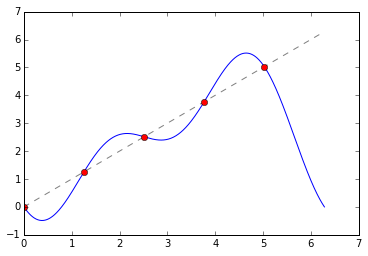# Fourier Interpolation

In :
import numpy as np
import numpy.linalg as la
import matplotlib.pyplot as pt


Let's fix the number of points and the number of functions as n.

Make sure n is odd.

In :
n = 5

assert n % 2 == 1

In :
x = np.linspace(0, 2*np.pi, n, endpoint=False)


Next, fix the values of $k$ in $\cos(kx)$ as cos_k and in $sin(kx)$ as sin_k so that there are exactly $n$ altogether:

In :
cos_k = np.arange(0, n//2 + 1, dtype=np.float64)
sin_k = np.arange(1, n//2 + 1, dtype=np.float64)

In :
print(cos_k)
print(sin_k)

[ 0.  1.  2.]
[ 1.  2.]



Next, build the generalized Vandermonde matrix.

Make sure to order the matrix by increasing $k$:

In :
V = np.zeros((n,n))
V[:, ::2] = np.cos(cos_k*x[:, np.newaxis])
V[:, 1::2] = np.sin(sin_k*x[:, np.newaxis])
V

Out:
array([[ 1.        ,  0.        ,  1.        ,  0.        ,  1.        ],
[ 1.        ,  0.95105652,  0.30901699,  0.58778525, -0.80901699],
[ 1.        ,  0.58778525, -0.80901699, -0.95105652,  0.30901699],
[ 1.        , -0.58778525, -0.80901699,  0.95105652,  0.30901699],
[ 1.        , -0.95105652,  0.30901699, -0.58778525, -0.80901699]])


Now let's try and do interpolation with this. Here are a few functions:

In :
if 1:
def f(x):
return x
elif 0:
def f(x):
return np.abs(x-np.pi)
elif 1:
def f(x):
return (x<=np.pi).astype(np.int32).astype(np.float64)


Find the coefficients as coeffs:

In :
coeffs = la.solve(V, f(x))

In :
plot_x = np.linspace(0, 2*np.pi, 1000)

In :
interpolant = 0 * plot_x
for i, k in enumerate(cos_k):
interpolant += coeffs[2*i] * np.cos(k * plot_x)
for i, n in enumerate(sin_k):
interpolant += coeffs[2*i+1] * np.sin(n * plot_x)

In :
pt.plot(plot_x, interpolant)
pt.plot(plot_x, f(plot_x), "--", color="gray")
pt.plot(x, f(x), "or")

Out:
[<matplotlib.lines.Line2D at 0x7f9157556470>]• For $f(x)=x$, why does it do what it does at the interval end?
• What happens if we increase the number of points?
• What if the function is actually periodic (e.g. the function with abs above?)?
• What if the function has jumps?
In :
# Answers
#
# * Because we're interpolating with periodic functions--so the interpolant is forced to be periodic.
# * We observe a distinct "overshoot". This overshoot is called "Gibbs phenomenon".
# * Periodic: no Gibbs at interval end.
# * Gibbs can also happen in the middle of the interval.


## Computing the coefficients

In :
B = V.T.dot(V)

In :
B[np.abs(B)<1e-12] = 0

In :
B

Out:
array([[ 5. ,  0. ,  0. ,  0. ,  0. ],
[ 0. ,  2.5,  0. ,  0. ,  0. ],
[ 0. ,  0. ,  2.5,  0. ,  0. ],
[ 0. ,  0. ,  0. ,  2.5,  0. ],
[ 0. ,  0. ,  0. ,  0. ,  2.5]])

• What do you observe?
• How could this be useful for computing the inverse?
• What is the normalization?
• This is a pretty special matrix. What is the cost of computing $Ax$ with it?
• The so-called Fast Fourier transform (FFT) computes a version of $Ax$ (with complex exponentials) in $O(n\log n)$!
In :
# Answers:
#
# * V's columns are orthogonal. (though not normalized)
# * The transpose of $V$ (with appropriate normalization) its inverse. This makes Fourier coefficients cheap to compute.
# * The normalization is n for the first entry, and n/2 for all the ones after that.
# * Computing Ax costs n**2 operations.